Function Repository Resource:

# TupperCipher

Encrypt with Tupper’s self-referential formula

Contributed by: Aster Ctor
 ResourceFunction["TupperCipher"][str,p] encrypts the string str with the prime p as the key. ResourceFunction["TupperCipher"][img,p] encrypts the image img with the prime p as the key.

## Details and Options

Tupper’s self-referential formula is a formula that visually represents itself when graphed at a specific location in the xy-plane.
The formula was defined by Jeff Tupper and appears as an example in Tupper's 2001 SIGGRAPH paper on reliable two-dimensional computer graphing algorithms.
ResourceFunction["TupperCipher"] encodes an image into an integer k according to this principle, so that the solution of the equation in the region k+p is this image.

## Examples

### Basic Examples (2)

Encrypt a string as input:

 In:=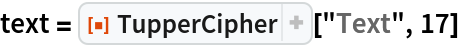Out=Test decoding via the resource function TupperDecipher:

 In:=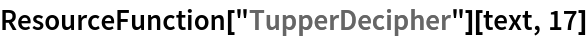Out=### Scope (3)

Define an image:

 In:=Out=Encrypt it:

 In:=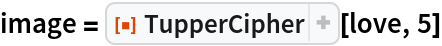Out=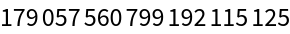Use the resource function TupperDecipher to test decoding:

 In:=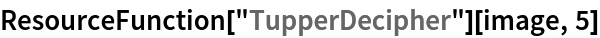Out=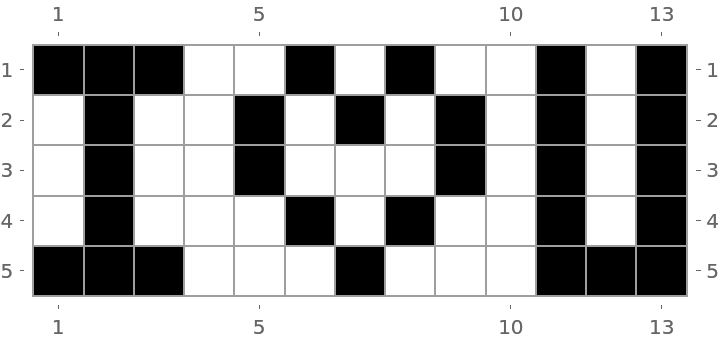## Publisher

Aster Ctor (MoeNet)

## Version History

• 1.0.0 – 26 December 2019